Archive for the ‘Math’ Category.

## The Blended Game

My PRIMES STEP students invented several variations of Penney’s game. We posted a paper about these new games at math.HO arxiv:2006.13002.

In Penney’s game, Alice selects a string of coin-flip outcomes of length n. Then Bob selects another string of outcomes of the same length. For example, Alice chooses HHT, and Bob chooses THH. Then a fair coin is tossed until Alice’s or Bob’s string appears. The player whose string appears first wins. In our example, Bob has a greater probability of winning, namely, 3/4. If the first two flips are HH, then Alice wins; otherwise, Bob wins.

The reader can check that HHT beats HTT with 2 to 1 odds. Thus, the game contains a non-transitive cycle it is famous for: THH beats HHT beats HTT beats TTH beats THH.

I already wrote about the No-Flippancy game that my students invented. It starts with Alice and Bob choosing different strings of tosses of the same length.

However, in the No-Flippancy game, they don’t flip a coin but select a flip outcome deterministically according to the following rule: Let in be the maximal length of a suffix in the sequence of “flips” that coincides with a prefix of the current player’s string. The player then selects the element of their string with index i + 1 as the next “flip.” Alice goes first, and whoever’s string appears first in the sequence of choices wins.

My favorite game among the invented games is the Blended game, which mixes the No-Flippancy game and Penney’s game.

In the game, they sometimes flip a coin and sometimes don’t. Alice and Bob choose their strings as in Penney’s game and the No-Flippancy game. Before each coin flip, they decide what they want by the rule of the No-Flippancy game above. If they want the same outcome, they get it without flipping a coin. If they want different outcomes, they flip a coin. Whoever’s string appears first in the sequence of `flips’ wins.

For example, suppose Alice selects HHT, and Bob selects THH. Then Alice wants H and Bob wants T, so they flip a coin. If the flip is T, then they both want Hs, and Bob wins. If the first flip is H, they want different things again. I leave it to the reader to see that Bob wins with probability 3/4. For this particular choice of strings, the odds are the same as in Penney’s game, but they are not always the same.

This game has a lot of interesting properties. For example, similar to Penney’s game, it has a non-transitive cycle of choices. Surprisingly, the cycle is of length 6: THH beats HHT beats THT beats HTT beats TTH beast HTH beat THH.

Share:

## The No-Flippancy Game

My STEP students invented a coin-flipping game that doesn’t require a coin. It is called The No-Flippancy Game.

Alice and Bob choose distinct strings of length n consisting of the letters H (for heads) and T (for tails). The two players alternate selecting the outcome of the next “flip” to add to the sequence by the rule below.

The “flip” rule: Let i < n be the maximal length of a suffix of the sequence of chosen outcomes that coincides with a prefix of the current player’s string. The player then selects the element of their string with index i + 1 as the next term in the sequence.

Alice goes first, and whoever’s string appears first in the sequence of choices wins. In layman terms, the game rules mean that the players are not strategizing, but rather greedily finishing their strings.

Suppose n = 2 and Alice chose HH. If Bob chooses HT, then Bob wins. Alice has to choose H for the first flip. Then Bob chooses T and wins. On the other hand, if Bob chooses TT for his string, the game becomes infinite. On her turn, Alice always chooses H, while on his turn Bob always chooses T. The game outcome is an alternating string HTHTHT… and no one wins.

Suppose n = 4, Alice chooses HHTT, and Bob chooses THHH. The game proceeds as HTHHTHHH, at which point Bob wins.

This game is very interesting. The outcome depends on how Alice’s and Bob’s chosen strings overlap with each other. We wrote a paper about this game, which is available at math.CO arXiv:2006.09588.

Share:

## Anchored Rectangles

Suppose we want to pack a unit square with non-overlapping rectangles that have sides parallel to the axes. The catch is that the lower left corners of all the rectangles are given. By the way, such rectangles are called anchored. Now, given some points in the unit square, aka the lower left corners, we want to find anchored rectangles with the maximum total area.

When the given points are close to the right upper corner of the square, the total area is small. When a single point is in the bottom left corner of the square, we can cover the whole square. The problem becomes more interesting if we add one extra assumption: one of the given points has to be the bottom left corner of the square. In the 1960’s, it was conjectured by Allen Freedman that any set of points has an anchored rectangle packing with the area of at least one half. The problem is quite resistant. In 2011, Dumitrescu and Tóth showed that every set of points has a packing of area at least 0.09, which was the first constant bound found, and is the best bound currently known.

I gave this problem to my PRIMES student Vincent Bian. He wrote a paper, Special Configurations in Anchored Rectangle Packings, that is now available at the arxiv. When you look at this problem you see that the number of ways to pack depends on the relative coordinates of the points. That means you can view the points as a permutation. Vincent showed that the conjecture is true for several different configurations of points: increasing, decreasing, mountain, split layer, cliff, and sparse decreasing permutations.

An increasing permutation is easy. There are two natural ways to pack the rectangles. One way, when rectangles are horizontal and each rectangle reaches to the right side of the square (see picture above). Another way, when rectangles are vertical. When you take the union of both cases, the square is completely covered, which means at least one of the cases covers at least half of the square. The worst case scenario, that is, the case when the maximum possible area is the smallest is when your points are placed equidistantly on the diagonal.

Other cases are more difficult. For example, Vincent showed that for a decreasing permutation with n points, the worst case scenario is when the points are arranged equidistantly on a hyperbola xy = (1-1/n)n. The picture shows the configuration for 15 points. The total area is more than one half.

Share:

## Happy 2019!

Happy 2019, the first 4 digit number to appear 6 times in the decimal expansion of Pi.

By the way:

2019 = 14 + 24 + 34 + 54 + 64.

Also, 2019 is the product of two primes 3 and 673. The sum of these two prime factors is a square.

This is not all that is interesting about factors of 2019. Every concatenation of these two prime factors is prime. Even more unusual, 2019 is the largest known composite number such that every concatenation of its prime factors is prime. [Oops, the last statement is wrong, Jan 3,2019]

Happy Happy-go-Lucky year, as 2019 is a Happy-go-Lucky number: the number that is both Happy and Lucky.

In case you are wondering, here is the definition of Happy numbers: One can take the sum of the squares of the digits of a number. Those numbers are Happy for which iterating this operation eventually leads to 1.

In case you are wondering, to build the Lucky number sequence, start with natural numbers. Delete every second number, leaving 1, 3, 5, 7, 9, 11, 13, 15, 17, 19, 21, …. The second number remaining is 3, so delete every third number, leaving 1, 3, 7, 9, 13, 15, 19, 21, …. The next number remaining is 7, so delete every 7th number, leaving 1, 3, 7, 9, 13, 15, 21, …. The next number remaining is 9, so delete every ninth number, etc.

Share:

## Two Dice

My friend Alex Ryba uses interesting math questions in the CUNY Math Challenge. For the 2016 challenge they had the following problem.

Problem. Eve owns two six-sided dice. They are not necessarily fair dice and not necessarily weighted in the same manner. Eve promises to give Alice and Bob each a fabulous prize if they each roll the same sum with her dice. Eve wishes to design her two dice to minimize the likelihood that she has to buy two fabulous prizes. Can she weight them so that the probability for Alice and Bob to get prizes is less than 1/10?

The best outcome for Eve would be if she can weight the dice so that the sum is uniform. In this case the probability that Alice and Bob get the prizes is 1/11. Unfortunately for Eve, such a distribution of weight for the dice is impossible. There are many ways to prove it.

I found a beautiful argument by Hagen von Eitzen on the stack exchange: Let ai (correspondingly bi) be the probabilities that die A (correspondingly B) shows i + 1. It would be very useful later that that i ranges over {0,1,2,3,4,5} for both dice. Let f(z) = ∑ aizi and g(z) = ∑ bizi. Then the desired result is that f(z)g(z) = ∑j=010 zj/11. The roots of the right side are the non-real roots of unity. Therefore both f and g have no real roots. So, they must both have even degree. This implies a5=b5=0 and the coefficient of z10 in their product is also 0, contradiction.

Alex himself has a straightforward argument. The probabilities of 2 and 12 have to be equal to 1/11, therefore, a0b0 = a5b5 = 1/11. Then the probability of a total 7 is at least a0b5 + a0b5. The geometric mean of a0b5 and a0b5 is 1/11 (from above), so their arithmetic mean is at least 1/11 and their sum is at least 2/11. Therefore, the uniform distribution for sums is impossible.

So 1/11 is impossible, but how close to it can you get?

Share:

## 3-Inflatable Permutations

We can inflate one permutation with another permutation. Let me define the inflation by examples and pictures. Suppose we have a permutation 132 which we want to inflate using permutation 21. The result is the permutation 216543 that can be divided into three blocks 21|65|43. The blocks are ordered as the first permutation 132, and within each block the order is according to the second permutation. This operation is often called a tensor product of two permutations. The operation is non-commutative: the inflation of 21 with 132 is 465132.As one might guess this post is related to k-symmetric permutations, that is, permutations that contain all possible patterns of size k with the same frequency. As I mentioned in my recent post 3-Symmetric Permutations, the smallest non-trivial examples of 3-symmetric permutations are 349852167 and 761258943 in size 9.

A permutation is called k-inflatable if its inflation with k-symmetric permutation is k-symmetric. One of my PRIMES projects was about 3-inflatable permutations. The result of this project is the paper On 3-Inflatable Permutations written with Eric Zhang and posted at the arxiv.

The smallest non-trivial examples of 3-inflatable permutations are in size 17: E534BGA9HC2D1687F and B3CE1H76F5A49D2G8, where capital letters denote numbers greater than nine. Another cool property discovered in the paper is that the tensor product of two k-inflatable permutations is k-inflatable.Share:

## Symmetries of k-Symmetric Permutations

I am fascinated by 3-symmetric permutations, that is, permutations that contain all possible patterns of of size three with the same frequency. As I mentioned in my recent post 3-Symmetric Permutations, the smallest non-trivial examples are in size 9.

When I presented these examples at a combinatorics pre-seminar, Sasha Postnikov suggested to draw the permutations as a graph or a matrix. Why didn’t I think of that?

Below are the drawings of the only two 3-symmetric permutations of size 9: 349852167 and 761258943.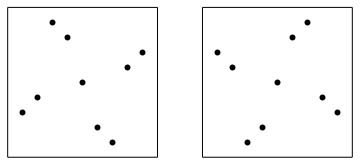As I already mentioned in the aforementioned essay the set of 3-symmetric permutations is invariant under the reversal and subtraction of each number from the size of the permutation plus 1. In geometrical terms it means reflection along the vertical midline and central symmetry. But as you can see the pictures are invariant under 90 degree rotation. Why?

What I forgot to mention was that the set of k-symmetric permutations doesn’t change after the inversion. In geometrical terms it means the reflection with respect to the main diagonal. If you combine a reflection with respect to a diagonal with a reflection with respect to a vertical line you get a 90 degree rotation. Overall, the symmetries of the k-symmetric permutations are the same as all the symmetries of a square. Which means we can only look at the shapes of the k-symmetric permutations.

There are six 2-symmetric permutations: 1432, 2341, 2413, 3142, 3214, 4123. As we can see in the picture below they have two different shapes.Here is the list of all 22 2-symmetric permutations of size 5: 14532, 15342, 15423, 23541, 24351, 24513, 25143, 25314, 31542, 32451, 32514, 34152, 34215, 35124, 41352, 41523, 42153, 42315, 43125, 51243, 51324, 52134. The list was posted by Drake Thomas in the comments to my essay. Up to symmetries the permutations form four groups. Group 1: 14532, 15423, 23541, 32451, 34215, 43125, 51243, 52134. Group 2: 15342, 24351, 42315, 51324. Group 3: 24513, 25143, 31542, 32514, 34152, 35124, 41523, 42153. Group 4: 25314, 41352. The picture shows the first permutation in each group.## 3-Symmetric Graphs

In my previous post I described 3-symmetric permutations. Now I want to define 3-symmetric graphs.

A reminder: a k-symmetric permutation is such that the densities of all permutations of size k in it are the same. In particular a 2-symmetric permutation has the same number of inversions and non-inversions. How do we translate this to graphs? I call a graph 2-symmetric if it has the same number of edges as non-edges. 2-symmetric graphs are easy to construct, but they only exist if the number of vertices, n, is such that n choose 2 is even. The simplest non-trivial examples are graphs with 4 vertices and three edges.

The above definition is difficult to generalize. So I rephrase: a graph G is 2-symmetric, if the density of any subgraph H with 2 vertices in G is the same as the expected density of H in a random graph where the probability of an edge equals 1/2. This definition is easy to generalize. A graph G is k-symmetric, if the density of any subgraph H with k vertices in G is the same as the expected density of H in a random graph where the probability of an edge equals 1/2. In particular, here are the densities of all four possible subgraphs with 3 vertices in a 3-symmetric graph:

• A complete graph with 3 vertices: 1/8,
• A path graph with 3 vertices: 3/8,
• A graph with 3 vertices and only one edge: 3/8,
• A graph with 3 isolated vertices: 1/8.

For a graph G to be 3-symmetric, the number of vertices, n, in G needs to be such that n choose 3 is divisible by 8. The first non-trivial case is n = 8. Here are the pictures of two 3-symmetric graphs. The first one is a wheel, and the second one is its complement.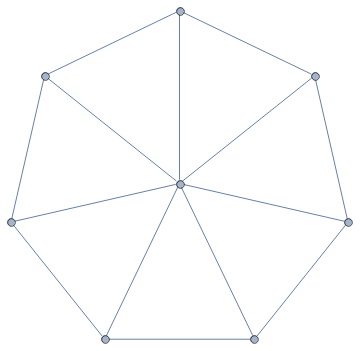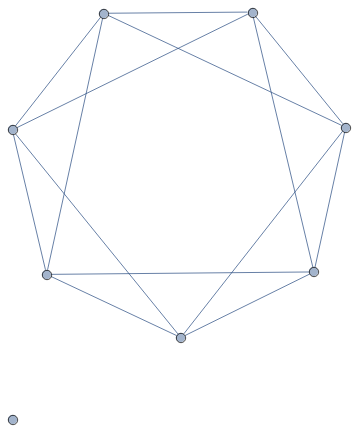## 3-Symmetric Permutations

I want to study patterns inside permutations. Consider a permutation of size 4: 1432. Today I am excited by ordered subset of size 3 inside this permutation. For example, I can drop the last number and look at 143. The ordering in 143 is the same as in 132, or, as a mathematician would say, 143 is order-isomorphic to 132. In my example of 1432, I have four subsets depending on which number I remove: 432 is order-isomorphic to 321, while 132, 142 and 143 are all order-isomorphic to 132.

The density of a pattern 123 in the permutation 1432 is the ratio of subsets of size 3 that are order-isomorphic to 123 to all subsets of size 3. As I already calculated, the density of 123 in 1432 is zero, while the density of 321 is 1/4, and the density of 132 is 3/4.

A permutation is called 3-symmetric if the densities of all patterns of size 3 (123, 132, 213, 231, 312, 321) are the same. The reason I love this notion, is that 3-symmetric permutations are similar to random permutations with respect to patterns of size 3.

My example permutation, 1432, is not 3-symmetric. Thinking about it, no permutation of size 4 can be 3-symmetric. The number of subsets of size 3 is four, which is not divisible by 6.

I wanted to find 3-symmetric permutations. So the size n of the permutation needs to be such that n choose 3 is divisible by 6. The numbers with this property are easy to find. The sequence starts as 1, 2, 9, 10, 18, 20, 28, 29, 36, 37, 38, 45, 46. The sequence is periodic with period 36.

Any permutation of sizes 1 or 2 is 3-symmetric as all densities are zero. Duh!

The next interesting size is 9. My student, Eric Zhang, wrote a program and found that there are two 3-symmetric permutations of size 9: 349852167 and 761258943. These numbers are so cool!. First, they are reverses of each other. This is not very surprising: if a permutations is 3-symmetric, then its reverse must also be 3-symmetric. There is another property: the permutations are rotational symmetries of each other. That is, the sum of two digits in the same place is 10. You can see that rotating a 3-symmetric permutation produces a 3-symmetric permutation.

I decided to write a program to find 3-symmetric permutations of the next size: 10. There is none. I do not trust my programming skills completely, so adjusted my program to size 9 and got the same result as Eric. I trust Eric’s programming skills, so I am pretty sure that there are no 3-symmetric permutations of size 10. Maybe there are some 3-symmetric permutations in size 18.

Let’s find 2-symmetric permutations. These are permutations with the same number of ascends and descends inversions and non-inversions. For n to be the size of such permutation n choose 2 needs to be divisible by 2. That means n has to have remainder 0 or 1 modulo 4. The first nontrivial case is n = 4. There are six 2-symmetric permutations: 1432, 2341, 2413, 3142, 3214, 4123. We can also group them into reversible pairs: 1432 and 2341, 2413 and 3142, 3214 and 4123. If we look at rotational symmetry we get different pairs: 1432 and 4123, 2341 and 3214, 2413 and 3142.

You can try to find non-trivial 4-symmetric permutations. Good luck! The smallest nontrivial size is 33. Finding 5-symmetric permutations is way easier: the smallest nontrivial size is 28. The sequence of nontrivial sizes as a function of n is: 1, 4, 9, 33, 28, 165, 54, 1029, 40832, 31752, 28680, 2588680, 2162700, and so on. My computer crashed while calculating it.Share:

## Shapes of Symbols in Latin Squares

Once John Conway showed me a cute way to enumerate Latin squares of size 4, up to the movements of the plane. It was a joint result with Alex Ryba, which is now written in a paper Kenning KenKen.

For starters, I want to remind you that a Latin square of size n is an n by n table filled with integers 1 through n, so that every row and column doesn’t have repeated integers. KenKen is a game that John Conway likes, where you need to recover a Latin square given some information about it.

Let me start by describing a particular shape of four cells that one digit can occupy in a Latin square of size 4. There are only seven different shapes. To get to the beautiful result, we need to number these seven shapes in a particular order starting from zero. The shapes are shown below.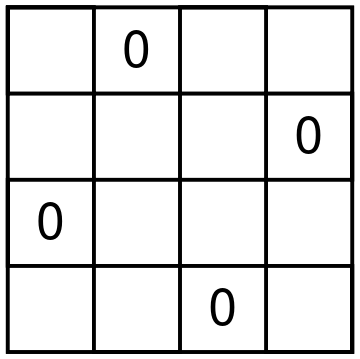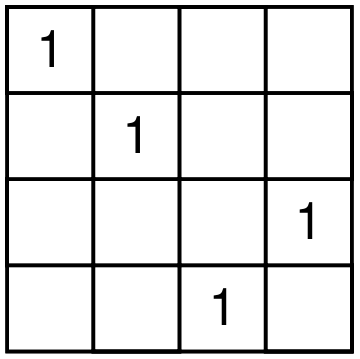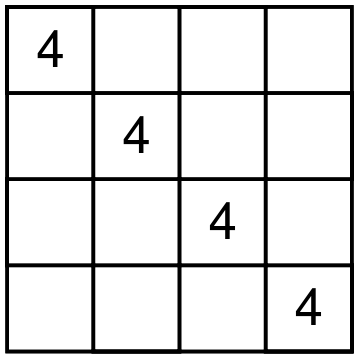There are 12 different Latin squares up to movements of the square and relabeling the digits. Here is how Conway and Ryba matches shapes and squares. For each Latin square, take the shapes of all four digits, remove the duplicate shape numbers and sum the leftover shape numbers. You will get a unique number from 1 to 12 that represents a particular Latin square. For example, consider the square on the picture below.Digit 1 is shape 4, digits 2 and 4 form shape 2, and digit 3 forms shape 6. Shape 2 is used twice, and we ignore multiplicities. So we have shapes 2, 4, and 6 used. The resulting Latin square is number 2 + 4 + 6, that is 12. It is a fun exercise to try to find all the squares. For example, square 1 can only use shapes 0 and 1. But shape 1 uses exactly one corner. So the first square should use each of the digits in shape 1.

John likes finding interesting ways to remember which shape is which. You can find his and Alex’s suggestions in the paper which Alex submitted to the arxiv.